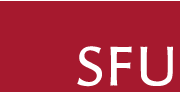# Algebraic and Arithmetic Geometry Research Group

## Our Research

Both algebraic and arithmetic geometry are concerned with the study of solution sets of systems of polynomial equations. Algebraic geometry deals primarily with solutions lying in an algebraically closed field, while arithmetic geometry deals with the more subtle study of solutions lying in a number field or its rings of integers. Both these topics are central to mathematics— and connect to subjects ranging from Number Theory and cryptography to mirror symmetry. Our individual research interests at SFU cover a broad spectrum, including toric geometry, linear subspaces of varieties, modular curves, and rational points on hyperelliptic curves.

The algebraic and arithmetic geometry group runs a regularly meeting research seminar. For more information, see link here...

## People

### Nils Bruin

Hyperelliptic curves, rational points, Chabauty methods, covering techniques, descent, local-to-global obstructions

### Imin Chen

Algebraic number theory, arithmetic geometry, representation theory, modular varieties, automorphic forms, diophantine problems, Galois representations, elliptic curves, Q-curves, function fields

### Nathan Ilten

Toric geometry, Fano varieties, mirror symmetry, deformation theory, linear subspaces of varieties, algebraic complexity theory

### Jake Levinson

Representation theory, Schubert calculus, moduli of curves, syzygies

### Postdoctoral Fellows and Visitors

• None Currently